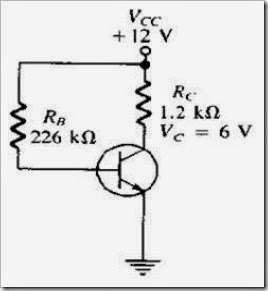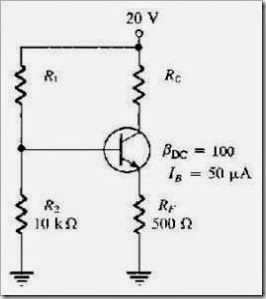# MCQs in Transistor Bias Circuits

(Last Updated On: December 8, 2017)

This is the Multiple Choice Questions in Transistor Bias Circuits from the book Electronic Devices – Electron Flow Version and Conventional Current Version 8th Edition by Thomas L. Floyd. If you are looking for a reviewer in Electronics Engineering this will definitely help. I can assure you that this will be a great help in reviewing the book in preparation for your Board Exam. Make sure to familiarize each and every questions to increase the chance of passing the ECE Board Exam.

### Online Questions and Answers Topic Outline

• MCQs in Transistor Bias Circuits
• MCQs in The DC Operating Point
• MCQs in Voltage-Divider Bias
• MCQs in Other Bias Methods
• MCQs in GreenTech Application 5: Wind Power

### Practice Exam Test Questions

Choose the letter of the best answer in each questions.

1. Voltage-divider bias has a relatively stable Q-point, as does

• A) base bias.
• B) collector-feedback bias.
• C) both of the above
• D) none of the above

2. Emitter bias requires

• A) only a positive supply voltage.
• B) only a negative supply voltage.
• C) no supply voltage.
• D) both positive and negative supply voltages.

3. Clipping is the result of

• A) the input signal being too large.
• B) the transistor being driven into saturation.
• C) the transistor being driven into cutoff.
• D) all of the above

4. Changes in βDC result in changes in

• A) IC.
• B) VCE.
• C) the Q-point.
• D) all of the above
5. The input resistance at the base of a voltage-divider biased transistor can be neglected
• A) at all times.
• B) only if the base current is much smaller than the current through R2 (the lower bias resistor).
• C) at no time.
• D) only if the base current is much larger than the current through R2 (the lower bias resistor).
6. What is the Q-point for a fixed-bias transistor with IB = 75 µA, βDC = 100, VCC = 20 V, and RC = 1.5 kΩ?
• A) VC = 0 V
• B) VC = 20 V
• C) VC = 8.75 V
• D) VC = 11.25 V
7. Ideally, for linear operation, a transistor should be biased so that the Q-point is
• A) near saturation.
• B) near cutoff.
• C) where IC is maximum.
• D) halfway between cutoff and saturation.

8. Refer to Figure 5-1. The value of IB isFigure 5-1

• A) 53 µA.
• B) 50 µA.
• C) 50 mA.
• D) 53 mA.

9. Refer to Figure 5-1. The value of IC is

• A) 10 µA.
• B) 10 mA.
• C) 5 mA.
• D) 50 mA.

10. Refer to Figure 5-1. The value of βDC is

• A) 5.3.
• B) 53.
• C) 94.
• D) 100.

11. Refer to Figure 5-2. Determine IC.Figure 5-2

• A) 5 µA
• B) 5 mA
• C) 0 mA
• D) 10 mA

12. Refer to Figure 5-2. Assume that IC ≈ IE. Find VE.

• A) 5 V
• B) 10 V
• C) 15 V
• D) 2.5 V
13. Refer to Figure 5-2. Assume IC ≈ IE. Determine the value of RC that will allow VCE to equal 10 V.
• A) 1 kΩ
• B) 1.5 kΩ
• C) 2 kΩ
• D) 2.5 kΩ

14. Refer to Figure 5-2. Calculate the current I2.

• A) 32 mA
• B) 3.2 mA
• C) 168 µA
• D) 320 µA
15. Refer to Figure 5-3(a). The most probable cause of trouble, if any, from these voltage measurements would be
• A) the base-emitter junction is open.
• B) RE is open.
• C) a short from collector to emitter.
• D) no problems.
16. Refer to Figure 5-3(b). The most probable cause of trouble, if any, from these voltage measurements is
• A) the base-emitter junction is open.
• B) RE is open.
• C) a short from collector to emitter.
• D) no problems.
17. Refer to Figure 5-3(c). The most probable cause of trouble, if any, from these voltage measurements is
• A) the base-emitter junction is open.
• B) RE is open.
• C) a short from collector to emitter.
• D) no problems.
18. Refer to Figure 5-3(d). The most probable cause of trouble, if any, from these voltage measurements is
• A) the base-emitter junction is open.
• B) RE is open.
• C) a short from collector to emitter.
• D) no problems.

19. The most stable biasing technique used is the

• A) voltage-divider bias.
• B) base bias.
• C) emitter bias.
• D) collector bias.

20. At saturation the value of VCE is nearly _____, and IC = _____.

• A) zero, zero
• B) VCC, IC(sat)
• C) zero, I(sat)
• D) VCC, zero
21. The linear (active) operating region of a transistor lies along the load line below _____ and above _____.
• A) cutoff, saturation
• B) saturation, cutoff

22. What is the most common bias circuit?

• A) base
• B) collector
• C) emitter
• D) voltage-divider

23. What is the dc input resistance at the base of a BJT?

• A) βDCRC
• B) βdc • (RC || RE)
• C) βDC • re′
• D) βDCRE
24. Which transistor bias circuit provides good Q-point stability with a single-polarity supply voltage?
• A) base bias
• B) collector-feedback bias
• C) voltage-divider bias
• D) emitter bias
25. Which transistor bias circuit arrangement has poor stability because its Q-point varies widely with βDC?
• A) base bias
• B) collector-feedback bias
• C) voltage-divider bias
• D) emitter bias
26. Which transistor bias circuit arrangement provides good Q-point stability, but requires both positive and negative supply voltages?
• A) base bias
• B) collector-feedback bias
• C) voltage-divider bias
• D) emitter bias
27. Which transistor bias circuit arrangement provides good stability using negative feedback from collector to base?
• A) base bias
• B) collector-feedback bias
• C) voltage-divider bias
• D) emitter bias
Refer to Figure 5-4. In the voltage-divider biased npn transistor circuit, if RC opens, the transistor is
• A) saturated.
• B) cutoff.
• C) nonconducting.
29. Refer to Figure 5-4. In the voltage-divider biased npn transistor circuit, if R2 opens, the transistor is
• A) saturated.
• B) cutoff.
• C) nonconducting.
30. Refer to Figure 5-4. In the voltage-divider biased npn transistor circuit, if R1 opens, the transistor is
• A) saturated.
• B) cutoff.
• C) nonconducting.